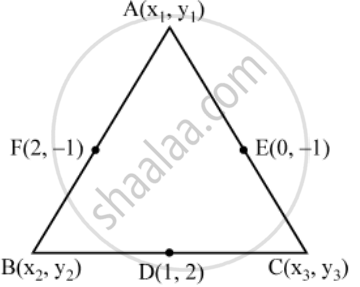Share

# If the coordinates of the mid-points of the sides of a triangle are (1, 2) (0, –1) and (2, 1). Find the coordinates of its vertices. - CBSE Class 10 - Mathematics

#### Question

If the coordinates of the mid-points of the sides of a triangle are (1, 2) (0, –1) and (2, 1). Find the coordinates of its vertices.

#### Solution

Let A(x_1 , y_1 ), B(x_2 , y_2 ) and C(x_3 , y_3 ) be the vertices of ∆ABC. Let D (1, 2), E (0, –1), and F(2, –1) be the mid-points of sides BC, CA and AB respectively. Since D is the mid-point of BC.

\therefore \(x_2+x_{3})/{2}=1\text{ and }(y_2+y_3)/{2}=2

⇒ x_2 + x_3 = 2 and y_2 + y_3 = 4 …. (1)

Similarly, E and F are the mid-point of CA and AB respectively.

\therefore (x_1+x_3)/{2}=0\text{ and }(y_1+y_3)/{2}=-1

⇒ x_1 + x_3 = 0 and y_1 + y_3 = – 2 …. (2)

(x_1+x_2)/{2}=2\text{ and }(y_1+y_2)/{2}=-1

⇒ x_1 + x_2 = 4 and y1 + y2 = –2 …. (3)

From (1), (2) and (3), we get

(x_2 + x_3 ) + (x_1 + x_3 ) + (x_1 + x_2 ) = 2 + 0 + 4 and, (y_2 + y_3 ) + (y_1 + y_3 ) + (y_1 + y_2 ) = 4 –2 – 2

⇒ 2(x_1 + x_2 + x_3 ) = 6 and 2(y_1 + y_2 + y_3 ) = 0 …. (4)

⇒ x_1 + x_2 + x_3 = 3 and y_1 + y_2 + y_3 = 0From (1) and (4), we get

x_1 + 2 = 3 and y_1 + 4 = 0

⇒ x_1 = 1 and y_1 = – 4

So, the coordinates of A are (1, – 4)

From (2) and (4), we get

x_2 + 0 = 3 and y_2 – 2 = 0

⇒ x_2 = 3 and y_2 = 2

So, coordinates of B are (3, 2)

From (3) and (4), we get

x_3 + 4 = 3 and y_3 – 2 = 0

⇒ x_3 = – 1 and y_3 = 2

So, coordinates of C are (–1, 2)

Hence, the vertices of the triangle ABC are A(1, – 4), B(3, 2) and C(–1, 2).

Is there an error in this question or solution?

#### Video TutorialsVIEW ALL 

Solution If the coordinates of the mid-points of the sides of a triangle are (1, 2) (0, –1) and (2, 1). Find the coordinates of its vertices. Concept: Section Formula.
S